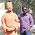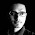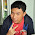Program to Solve System Of Linear Equations With Step By Step Solution

Four years ago when I was in my first year of B-Tech, I have written a program in C to solve for given system of linear equations. I actually wrote it for my personal use then. Once my maths lecturer gave a long assignment on solving system of linear equations. I did not feel like doing that. So, I wrote this program to solve the equations by generating a step by step solution (so that I can copy in the assignment) and also give information about all the related properties like rank of the matrix, nature of solution, etc. (If you are in a hurry, you can scroll down the page to quickly download the program)

Recently when I was programming for some other project, I was in need of that code. I've searched my hard disk for it but I could not find the source code which I have written long back. Although, luckily I have found the DOS executable in the sent folder in my mail-box. I've sent this program to all my friends then, that helped me retain it now. I wrote and compiled this using Turbo C. Along with this program, I have also recollected many other programs which I wrote long back. I will share all them with you soon in my upcoming posts.

Although the software is intuitive if you know the maths of it. However, let me explain its usage in brief.

This program was compiled for DOS. It should work well on any version of Windows that has DOS included. Linux or Mac users can use DOS emulators like DOSBox to run this program.

This program uses Gaussian Elimination method also called Gauss-Jordan Elimination method to solve the system of linear equations.

You can now double click on the downloaded file. The start screen should open as shown in the screen-shots below.

Now I will consider a problem to illustrate using this program.
For example if we are given the following set of linear equations:
2X+3Y-Z=-4
X-Y-2Z=-4
3X+Y+3Z=-2

If we represent the above equations in a general matrix form as
AX=B where
A is the coefficient matrix => A=[(2, 3, -1); (1, -1, -2); (3, 1, 3)]
X is the variable matrix => X= [X, Y, Z]
B is the constants matrix => B=[-4, -4, -2]

The matrix AB is called the augmented matrix. It is obtained by writing the matrix B as the last column of matrix A. => [AB] = [(2, 3, -1); (1, -1, -2); (3, 1, 3); (-4, -4, -2)]
We use this Augmented matrix to solve the set of linear equations.

Screenshot-1

Enter number of rows and columns of the augmented Matrix:

Screenshot-2

The input given is 3  4
By this, we are giving the input to the program that our augmented matrix has three rows and four columns

Screenshot-3

Next comes the screen on the command promt asking to enter the values of augmented matrix. Enter the values as shown in the screenshot.

Screenshot-4

Now the program displays the procedure step-by-step also showing the steps that are followed.

Screenshot-5

It also indicates the nature of solution for the given set of linear equations. i.e, if it has a unique solution or many solutions or no solution.

Screenshot-6

After doing a few more steps, we get the solution set. In this case we got the solution set as
{-1.76, 0.18, 1.03}
which means that X=-1.76, Y=0.18 and Z=1.03

I actually want the source code of this for a different purpose. I should check my other computer which is not presently with me to find this source code. If not I will write it again and share it with you soon.

You can download this program by clicking the links below. (File size 24KB zipped and 36KB unzipped)

It is to be free of any malware. Scanned by about 40 antivirus softwares using virus total.
You can freely use it and share it as you like.

1.Hello, I have read your comment in http://www.howtogeek.com/124831/ask-the-readers-what-are-your-must-have-presentation-tools/. You said that you use GIMP and Impress. I am a Linux user, I interested in new ideas about Linux. Let me look your presentation works.

2.Its very nice one. I like to this one as my final year project. Can You help me? I need the circuit. Please sent to my mail id.

1.Sure, send me your email ID, I will forward you the code.

3.This is nice I nid a program code in c++ that will solve this question.given that y=ax^7+bx^5-cx where a,b,c are integers.write a program to compute the value of y at any instance.............pleasemy mail is aosalami28@student.lautech.edu.ng......thanx so much

4.sir i want to create a project.the device which is used as tailor.means it takes input as a dimensions and cloth(already cut) and gives result as final dress.please give an idea how to create that.nandini.bvc@gmail.com

1.Hi Nandini, Thats a great idea. I think it can be implemented conveniently in Java or HTML5.
If you can explain me the calculations and dress designing procedure, I can help you in building it.
You can contact me for more.

5.can I have the code? please send it to me: HectorBL24@yahoo.com

6.sir, may i ask of the source code? i'm amazed with how you made it. jetron_adtoon@yahoo.com. thank you.